## Filters

Sort by :
Clear All
Q
Engineering
1585 Views   |

The correct decreasing order of priority for the functional groups of organic compounds in the IUPAC system of nomenclature is

• Option 1)

– CONH2 , – CHO, – SO3H , – COOH

• Option 2)

– COOH , – SO3H , – CONH2 , – CHO

• Option 3)

– SO3H , – COOH , – CONH2 , – CHO

• Option 4)

– CHO , – COOH ,– SO3H , – CONH2

Option 2 is correct

Engineering
470 Views   |

Which of the following factors is of no significance for roasting sulphide ores to the oxides and not subjecting the sulphide ores to carbon reduction directly?

• Option 1)

is more volatile than

• Option 2)

Metal sulphides are thermodynamically more stable than

• Option 3)

is thermodynamically more stable than

• Option 4)

Metal sulphides are less stable than the corresponding oxides

As we learnt in Roasting - It is heating of the ore in presence of air below its fusion temperature. - wherein 2PbS +3O3  2PbO + 2SO2   The reduction of metal sulphides by carbon reduction has positive   whereas,  is negative for the same process with metal oxides. So, metal sulphides are more stable than oxides.    Option 1) is more volatile than  This option is incorrect. Option 2) Metal...
Engineering
113 Views   |

The organic chloro compound, which shows complete stereochemical inversion during a reaction, is

• Option 1)

• Option 2)

• Option 3)

• Option 4)

As we learnt in  SN2 Substitution Nucleophilic bimolecular - These reactions proceed in only one step.    The only carbon atom in  undergoes inversion there by giving us complete stereochemical inversion in the  reaction. Option 1) Correct Option 2) Incorrect Option 3) Incorrect Option 4) Incorrect
Engineering
116 Views   |

• Option 1)

enantiomers

• Option 2)

conformers

• Option 3)

epimers

• Option 4)

anomers

As we learnt in Anomers - The two forms of glucose,   glucose and   glucose , are called anomers . Both are optically active and differ at   position .  - wherein Specific rotation of   glucose is  and of  glucose is     Anomers are optically active compounds. Option 1) enantiomers This option is incorrect Option 2) conformers This option is incorrect Option 3) epimers This option is...
Engineering
864 Views   |

Bakelite is obtained from phenol by reaction with

• Option 1)

• Option 2)

• Option 3)

• Option 4)

As we learnt in Phenol formaldehyde resin/ Bakellite/ Novolac - - Obtained by the condensation reaction of phenol with formaldehyde. - Step growth, Copolymer. - Novolac is linear - Bakellite is cross linked. - wherein - Hard and brittle - Novolac is used in paints. - Bakellite is used in making combs, phonograph records, electrical goods.     The polymerisation of phenol and formaldehyde...
Engineering
109 Views   |

In context with the industrial preparation of hydrogen from water gas , which of the following is the correct statement?

• Option 1)

is oxidised to with steam in the presence of a catalyst followed by absorption of in alkali

• Option 2)

and   are fractionally separated using differences in their densities

• Option 3)

is removed by absorption in aqueous  solution

• Option 4)

is removed through occlusion with Pd

As we learnt in Water Gas Shift Reaction - Reaction of with water at 673 K in presence of catalyst. - wherein     Option 1) is oxidised to with steam in the presence of a catalyst followed by absorption of in alkali This  option is correct Option 2) and   are fractionally separated using differences in their densities This  option is incorrect Option 3)   is removed by absorption in...
Engineering
345 Views   |

Identify the wrong statement in the following.

• Option 1)

Acid rain is mostly because of oxides of nitrogen and sulphur

• Option 2)

Chlorofluorocarbons are responsible for ozone layer depletion

• Option 3)

Greenhouse effect is responsible for global warming

• Option 4)

Ozone layer does not permit infrared radiation from the sun to reach the earth

As we learnt in Allotropes of Oxygen - O2 (Dioxygen) and O3 (Ozone) -   Infrared radiation is most important for heating up the atmosphere and the surface of the earth. These rays have high wavelength and low frequency and are not very harmful. Ozone does not hinder infrared radiation. Option 1) Acid rain is mostly because of oxides of nitrogen and sulphur This option is incorrect. Option...
Engineering
142 Views   |

Which one of the following is the correct statement?

• Option 1)

is known as ‘inorganic benzene’

• Option 2)

Boric acid is a protonic acid.

• Option 3)

Beryllium exhibits coordination number of six

• Option 4)

Chlorides of both beryllium and aluminium have bridged chloride structures in solid phase

As we learnt in  Property of aluminium chloride - Dimeric structure disappears when dissolved in water. It is hygroscopic in nature. -    displays octahedral coordination geometry.    Option 1)   is known as ‘inorganic benzene’ This option is incorrect. Option 2) Boric acid is a protonic acid. This option is incorrect. Option 3) Beryllium exhibits coordination number of six This option is...
Engineering
136 Views   |

Larger number of oxidation states are exhibited by the actinoids than those by the lanthanoids, the main reason being

• Option 1)

More reactive nature of the actinoids than the lanthanoids

• Option 2)

orbitals more diffused than the orbitals

• Option 3)

Lesser energy difference between and than between and   orbitals

• Option 4)

More energy difference between and than between and   orbitals

As we learnt in Oxidation states - The common oxidation state of lanthanoid is +3 low occasionally +2  and  +4  ions in solution or in the solid compound are also obtained. -    and Oxidation states - Ac shows +3 oxidation state generally but some Ac. Also, stable till +7 oxidation states. -    Actinoids exhibits more number of oxidation state in general than the Lanthanoids. This is because of...
Engineering
128 Views   |

Given

The potential for the cell

is

• Option 1)

• Option 2)

• Option 3)

• Option 4)

As we learnt in   Electrode Potential(Nerst Equation) - - wherein is concentration of species F= 96487 C  R= 8.314  T= Temperature in kelvin                      According to Nernst equation   Option 1) This option is incorrect. Option 2) This option is correct. Option 3) This option is incorrect. Option 4) This option is incorrect.
Engineering
162 Views   |

Amount of oxalic acid present in a solution can be determined by its titration with solution in the presence of . The titration gives unsatisfactory result when carried out in the presence of  , because

• Option 1)

oxidises oxalic acid to carbon dioxide and water

• Option 2)

gets oxidised by oxalic acid to chlorine

• Option 3)

Furnishes ions in addition to those from oxalic acid

• Option 4)

reduces permanganate to

As we learnt in Standard Electrode Potential Value - -     Oxalic acid present in a solution can be determined by its titration with solution in the presence of Titration cannot be done in the presence of because being a strong oxidizing agent oxidises   and get itself reduced to So actual amount of oxalic acid in solution can­ not be determined. Option 1) oxidises oxalic acid to carbon...
Engineering
132 Views   |

For a reaction rate of disappearance of is related to the rate of appearance of by the expression

• Option 1)

• Option 2)

• Option 3)

• Option 4)

As we learnt in Rates in presence of stoichiometry of reactants/products - When stoichiometry coefficients of reactants/ products are not equal to one, the rate of disappearance of  & the rate of appearance of products is divided by their respective stoichiometric coefficients - wherein     or Option 1) Incorrect Option Option 2) Incorrect Option Option 3) Correct Option Option...
Engineering
118 Views   |

Oxidising power of chlorine in aqueous solution can be determined by the parameters indicated below: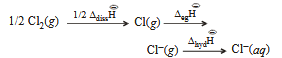The energy involved in the conversion of    (using data ,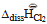= 240 kJ mol-1  ,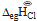= = -349 kJ mol-1,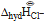= -381 kJ mol-1)   will be

• Option 1)

+120 kJ mol-1

• Option 2)

+152 kJ mol-1

• Option 3)

-610 kJ mol-1

• Option 4)

-850 kJ mol-1

As we learnt in Bond dissociation enthalpy - It is the average of enthalpy required to dissociate the said bond present in different gaseous compound in to free atoms in gaseous state. - wherein     Enthalpy of Hydration - Amount of enthalpy evolved during hydration of 1 mole anhydrous compound. - wherein    The energy involved in the conversion of to is given Substituting various values...
Engineering
99 Views   |

Standard entropy of   are 60, 40 and 50 J K-1 mol-1, respectively. For the reaction,

to be at equilibrium, the temperature will be

• Option 1)

1000 K

• Option 2)

1250 K

• Option 3)

500 K

• Option 4)

750 K

As we learnt in  Gibb's free energy (Δ G) -   - wherein Gibb's free energy enthalpy of reaction entropy temperature     Entropy for isothermal process - or   - wherein     For a reaction to be equilibrium  since  so at equilibrium  For reaction Calculating  for the above equation, we get  At equilibrium,  Option 1) 1000 K This option is incorrect  Option 2) 1250 K This option...
Engineering
122 Views   |

In the following sequence of reactions, the alkene affords the compound B

The compound B is

• Option 1)

• Option 2)

• Option 3)

• Option 4)

As we learnt in  Ozonolysis - Ozonolysis of alkenes involved the addition of ozone molecules to alkene to form ozonide and then cleavage of the ozonide by Zn - H2O to smalter molecules.This reaction is highly useful in detecting the position of the double bond in alkene or other unsaturated compounds. - wherein     Option 1) This option is correct. Option 2) This option is...
Engineering
303 Views   |

The hydrocarbon which can react with sodium in liquid ammonia is

• Option 1)

• Option 2)

• Option 3)

• Option 4)

As we learnt in  Birch Reduction - Alkynes on reduction with sodium in liquid NH3 form trans alkene. - wherein    Alkynes having terminal react with Na in liquid NH3 to yield H2. Option 1) This option is incorrect. Option 2) This option is incorrect. Option 3) This option is correct. Option 4) This option is incorrect.
Engineering
204 Views   |

Which one of the following constitutes a group of the isoelectronic species?

• Option 1)

• Option 2)

• Option 3)

• Option 4)

As we learnt in

Bond strength -

The energy required to break one mole of bonds of particular type in gaseous state is called bond energy or bond strength.

- wherein

The same amount of energy is released in formation of one mol of particular bond.

Number of electrons in each species are given below

It is quite evident from the above that are isoelectronic in nature.

Hence is correct

Option 1)

This option is incorrrect

Option 2)

This option is incorrrect

Option 3)

This option is corrrect

Option 4)

This option is incorrrect

View More
Engineering
385 Views   |

Which one of the following pairs of species have the same bond order?

• Option 1)

• Option 2)

• Option 3)

• Option 4)

As learnt in

Bond Order -

Bond order is defined as one half the difference between the number of electrons present in the bonding and the antibonding orbitals.

- wherein

In the given pair of species number of electron in = number of electron in electrons.

So they are isoelectronic in nature. Hence bond order of these two species will be also similar which is shown below.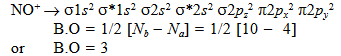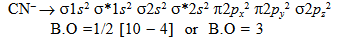NO+ and CN- has same bond order..

Option 1)

This option is incorrect

Option 2)

This option is correct

Option 3)

This option is incorrect

Option 4)

This option is incorrect

View More
Engineering
145 Views   |

Gold numbers of protective colloids A,B,C and D are 0.50, 0.01, 0.10 and 0.005, respectively. The correct order of their protective powers is

• Option 1)

• Option 2)

• Option 3)

• Option 4)

As we learnt in  Gold Number  Gold Number  Since Gold Number is  Hence Protective Power is  Option 1) This option is Incorrect.  Option 2) This option is Incorrect.  Option 3) This option is Incorrect.  Option 4) This option is Correct.
Engineering
152 Views   |

The ionization enthalpy of hydrogen atom is The energy required to excite the electron in the atom from

• Option 1)

• Option 2)

• Option 3)

• Option 4)

As we learnt in

Total energy of elctron in nth orbit -

Where z is atomic number

-

The ionisation of H­ atom is the energy absorbed when the electron in an atom gets excited from first shell (E1) to infinity

Energy of electron in second orbit ( n=2)

Energy required when an electron makes transition from  n =  1 to n = 2

Option 1)

Correct option

Option 2)

Incorrect option

Option 3)

Incorrect option

Option 4)

Incorrect option

View More
Exams
Articles
Questions